Statistical Distributions for the Random Function (MathScript RT Module)

LabVIEW 2012 MathScript RT模块帮助

»查看产品信息下载帮助（仅限Windows）

The following table describes the statistical distributions that the LabVIEW MathScript random function supports.

Distribution Equation Parameters
beta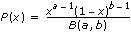for 0≤x≤1
a>0, b>0
binomial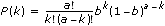for k=0, 1, ... , a
a is a positive integer; 0≤b≤1
chi-squared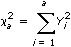where Yi have normal independent distributions with mean 0 and variance 1, and a is the degree of freedom.
a is a positive integer
exponential P(x) = aeax a>0
F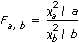where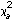and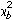have chi-square independent distributions with degrees of freedom a and b.
a and b are positive integers
gamma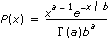a>0, b>0
geometric P(k) = a(1–a)k

for k=0, 1, 2, ...
0≤a≤1
hypergeometric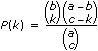where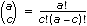.
a, b, and c are positive integers, ba, ca
lognormal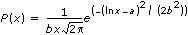a, b>0
negative binomial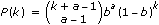for k=0, 1, 2, ...
a is a positive integer, 0≤b≤1
noncentral F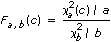where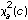andare two independently-distributed variables.
a and b are positive integers, c>0
noncentral T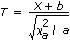where X andare two independently-distributed variables, X has a normal distribution, and a is the degree of freedom.
a is a positive integer, b
noncentral chi-square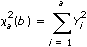where Yi have normal independent distributions with mean √(b/a) and variance 1, and a is the degree of freedom.
a is a positive integer, b>0
normal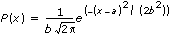a, b>0
Poisson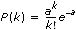for k=0, 1, 2, ...
a>0
Rayleigh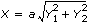where Yi have normal independent distributions with mean 0 and variance 1.
a>0
T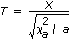where X andare two independently-distributed variables, X has a normal distribution, and a is the degree of freedom.
a is a positive integer
discrete uniform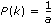for k = 1, 2, ..., a
a is a positive integer
continuous uniformfor a<x<b
a<b
Weibull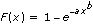a>0, b>0

﻿